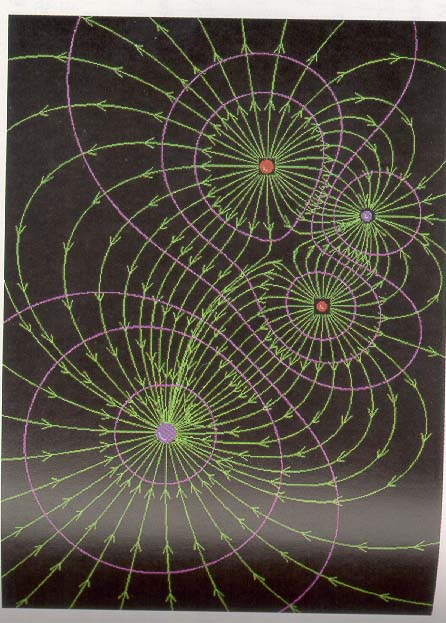Equipotential surfaces

Equipotential surfaces can be shown as lines in two dimensions to provide a quantitative way of viewing electric potential. Every point on a given line is at the same potential. Such maps can be read like topographic maps.

 For instance consider the map on the right of the Rawah Wilderness in northern Colorado . All points on the same line are at the same elevation, just as all points on the same equipotential line are at the same voltage. Water flows downhill, so the rivers are always perpendicular to the contour lines on the topographic map. This is similar to the way electric field lines are always perpendicular to equipotential lines. When contour lines are close together, the slope is steep, e.g. a cliff, just as close equipotential lines indicate a strong electric field. Lakes are at the same elevation, in the same way conductors are at the same potential.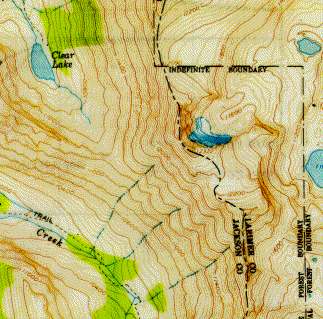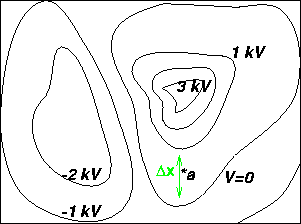The figure to the left shows equipotential lines. The electric field at point a can be found by calculating the slope at a: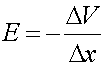where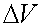is the voltage difference between the two lines near a, and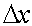is the distance between the two lines nearest a.

Rules for equipotential lines:

1. Electric field lines are perpendicular to the equipotential lines, and point "downhill": from higher potential toward lower.
2. A conductor forms an equipotential surface.
3. Where equipotential surfaces are close to each other, the electric field is strong.

Below are a set of electric field lines and equipotential surfaces caused by a certain set of point charges (taken from page 588 of the textbook). You can use it to test your skill at determining where the field is strong or weak on the basis of the density of field lines, or on the basis of how rapidly the potential is changing. Note that there should be an infinite number of equipotential surfaces surrounding each point charge; unfortunately their artist got tired of drawing them rather soon.